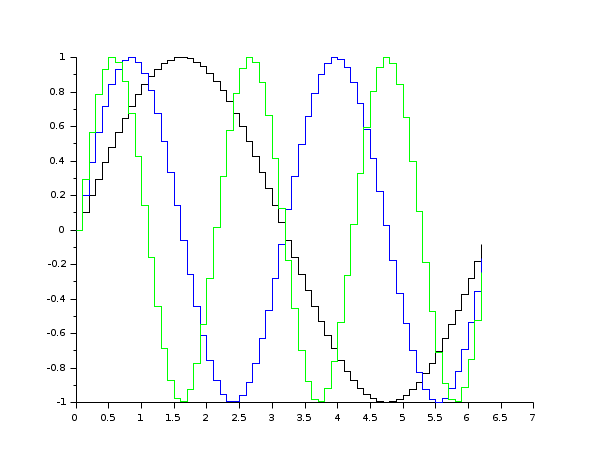Scilab Home page | Wiki | Bug tracker | Forge | Mailing list archives | ATOMS | File exchange
Change language to: Français - Português - 日本語 - Русский

See the recommended documentation of this function

Scilab Help >> Graphics > 2d_plot > plot2d2

# plot2d2

2D plot (step function)

### Calling Sequence

```plot2d2([x],y)
plot2d2([x],y,<opt_args>)
plot2d2([logflag],x,y,[style,strf,leg,rect,nax])```

### Arguments

args

see `plot2d` for a description of parameters.

### Description

`plot2d2` is the same as `plot2d` but the functions given by `(x,y)` are supposed to be piecewise constant.

By default, successive plots are superposed. To clear the previous plot, use `clf()`.

Enter the command `plot2d2()` to see a demo.Note that all the modes proposed by `plot2dxx` (xx = 1 to 4) can be enabled using `plot2d` and setting the `polyline_style` option to the corresponding number.

### Examples

```// plots a step function of value i on the segment [i,i+1]
// the last segment is not drawn
plot2d2([1:4],[1:4],1,"111","step function",[0,0,5,5])``````// compare the following with plot2d
x=[0:0.1:2*%pi]';
clf()
plot2d2(x,[sin(x) sin(2*x) sin(3*x)])``````x=[0:0.1:2*%pi]';
clf();
plot2d(x,[sin(x) sin(2*x) sin(3*x)])
e=gce();
e.children(1).polyline_style=2;
e.children(2).polyline_style=2;
e.children(3).polyline_style=2;```• plot2d — 2D plot
• plot2d3 — 2D plot (vertical bars)
• plot2d4 — 2D plot (arrows style)
• subplot — divide a graphics window into a matrix of sub-windows
• clf — Clear or reset or reset a figure or a frame uicontrol.
• polyline_properties — description of the Polyline entity properties